# 6. When a ray of light travels from one medium to another, it changes direction. This phenomenon isknown as refraction. In the figure below, light is traveling from A to B. The amount of refractiondepends on the velocities vi and v2, of light in the two media and on Fermat's Principle which statesthat the light's time of travel, T, from A to B, is a minimum(a) Find an expression for T in terms of x and the constants a, b, v1, v2 and c.(b) Show that if R is chosen so that the time of travel is minimized, thensin 01sin 02This result is known as Snell's Law and the ratio v1/v2 is called the index of refraction of thesecond medium with respect to the first medium. (Suggestion: Calculate set 0 but donot solve for x. Use this final equation to directly calculate the fraction Medium 1Velocity1A01RB'-C--Medium 2BVelocity 2CFigure 2: Snell's Law

Question
32 views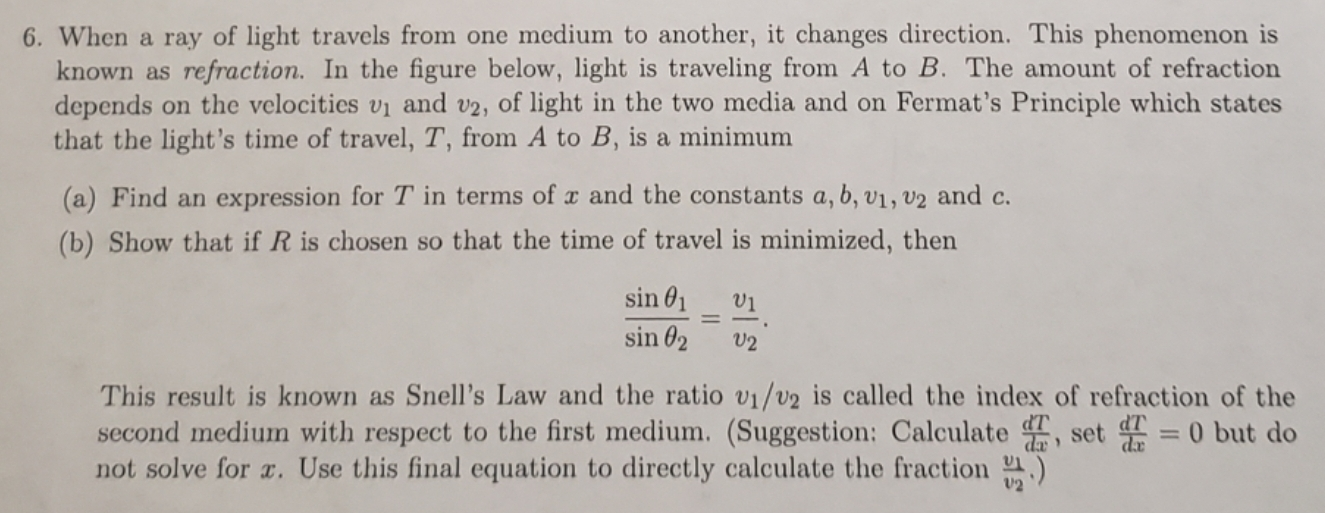help_outlineImage Transcriptionclose6. When a ray of light travels from one medium to another, it changes direction. This phenomenon is known as refraction. In the figure below, light is traveling from A to B. The amount of refraction depends on the velocities vi and v2, of light in the two media and on Fermat's Principle which states that the light's time of travel, T, from A to B, is a minimum (a) Find an expression for T in terms of x and the constants a, b, v1, v2 and c. (b) Show that if R is chosen so that the time of travel is minimized, then sin 01 sin 02 This result is known as Snell's Law and the ratio v1/v2 is called the index of refraction of the second medium with respect to the first medium. (Suggestion: Calculate set 0 but do not solve for x. Use this final equation to directly calculate the fraction fullscreen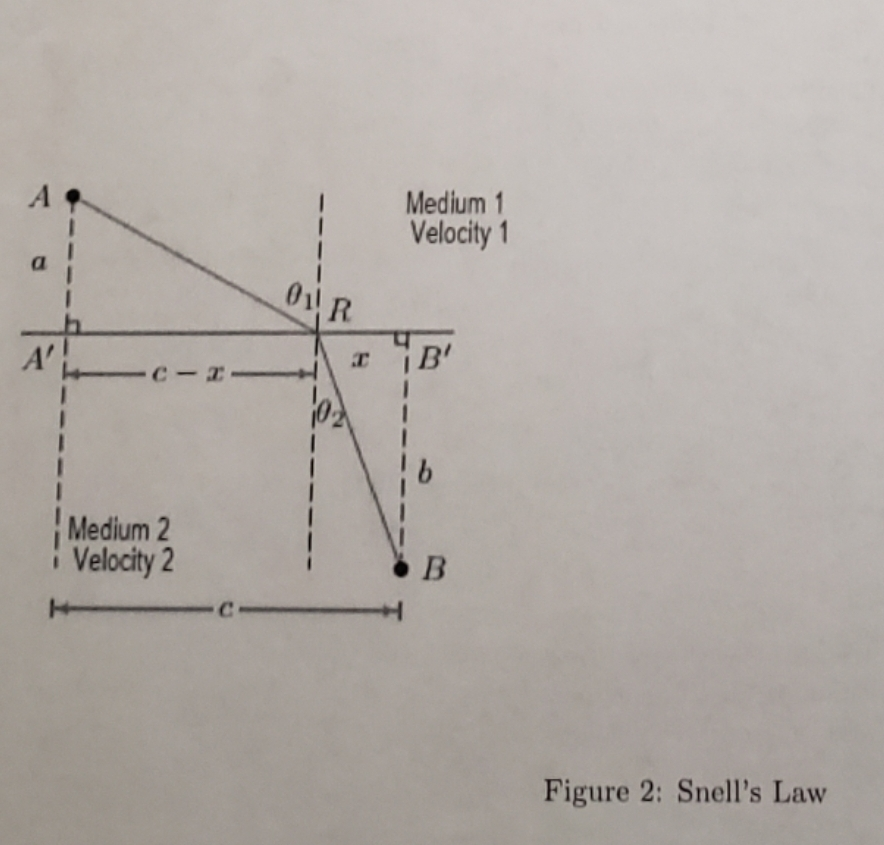help_outlineImage TranscriptioncloseMedium 1 Velocity1 A 01R B' -C-- Medium 2 B Velocity 2 C Figure 2: Snell's Law fullscreen
check_circle

Step 1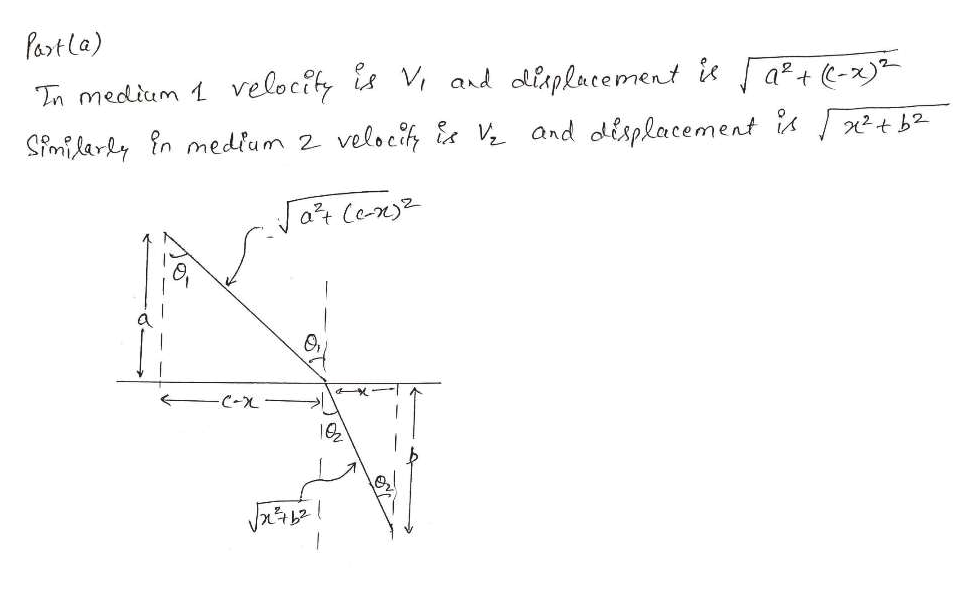help_outlineImage TranscriptionclosePart la) Tn medliam 1 velocity is V ard olisplacement ia-) Simidary n medium 2 veloc V and displacement i tb at Cen C-X fullscreen
Step 2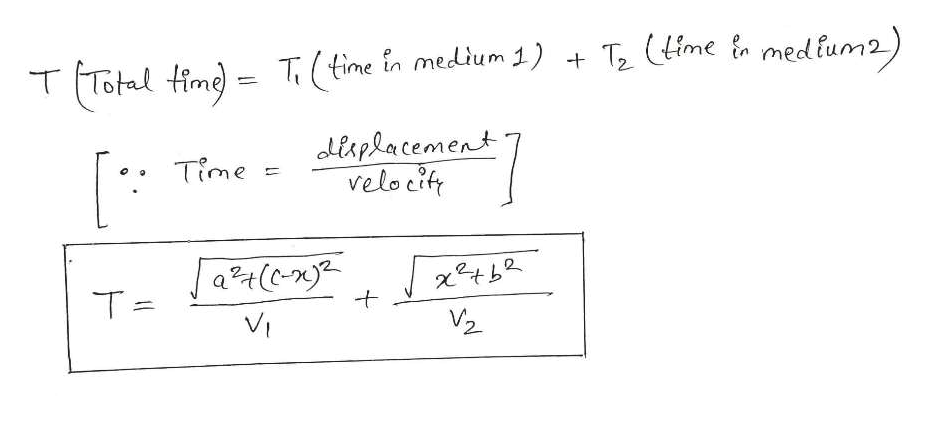help_outlineImage TranscriptioncloseT2 (ime med fum2) TTotal fime=T(time in medlum 1) + diaplacement velocit Time at(n T = VI fullscreen
Step 3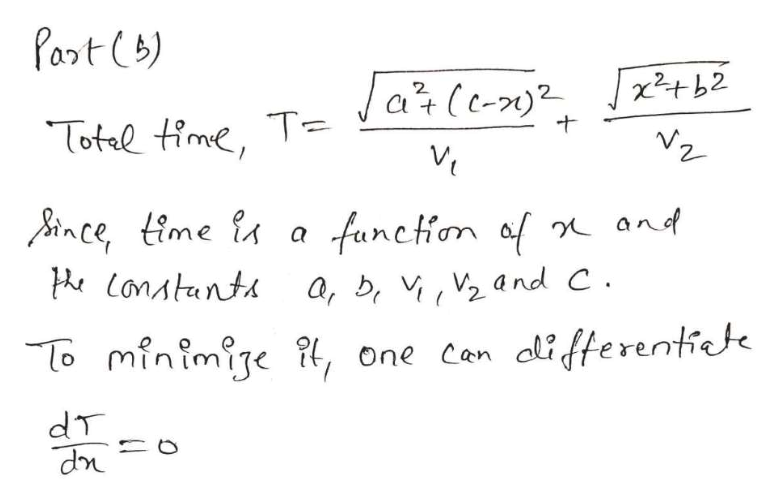help_outlineImage TranscriptionclosePart (8) x2 b2 Ja (e- 2. T= Totel time, VZ and a fanction af ince time Lonatants a, b V2and C o minimige , one can alifferentiete dT dn fullscreen

### Want to see the full answer?

See Solution

#### Want to see this answer and more?

Solutions are written by subject experts who are available 24/7. Questions are typically answered within 1 hour.*

See Solution
*Response times may vary by subject and question.
Tagged in

### Physics